# Chemical Kinetics

By: Colleen Tuttle

Abstract

Chemical kinetics determines the overall order of the reaction, as well as the order of each of the reactants.  In this lab, a series of reactions, with slight variations in concentrations were completed so the overall order of the reaction could be determined.

Introduction

Chemical kinetics is the study of determining the rate of a reaction under certain conditions.  The rate law of a reaction uses the kinetic information of the concentrations at various times of the reactants in the experiment.  The method of determining this rate of reaction used commonly is called Pseudo-Orders.  Pseudo-orders are based on the initial concentrations of the reactants, and the rate law is then determined experimentally, since the rate will be measured during the experiment.  The rate expression for pseudo-orders is given by R=k[A]x[B]y, where A and B are the reactants, x and y are the individual orders, and k is the rate constant.  X, y, and k can only be determined experimentally.  When determining the individual orders for the reactants, one of the concentrations must be kept constant.  If one was to determine the value of x, the concentration of reactant B would need to be kept constant, while the concentration of reactant A was changed, which would, in turn, also affect the rate.  These values are then inputted into the equation above and divided by one another.  By keeping the concentration of B constant, that term has been eliminated from the equation, as has the rate constant, k.  This leaves the equation as the R1/R2=([A]1/[A]2)x, and from this, y can be determined by taking the log of both sides of the equation, and then simple division.  Y is determined in the same manner, only by keeping the concentration of reactant A constant.  After x and y have determined, k can be calculated.  This is done by using each reaction and determining the individual k, then averaging all of them to determine the overall rate constant.  Using the same equation as above, k is determined from a simple algebraic equation of dividing the rate by the concentration of A taken to its order and the concentration of B taken to its order.  Simply written, it is k = R/([A]x[B]y). After all the individual rate constants have determined and averaged, the final rate law can be written.2

The quantities of x, y, and k can only be obtained through experimental data because they are empirical numbers.  Therefore, if these same reactions were done under different conditions than originally performed, the values may be different.  Temperature, concentrations of the reactants, impurities, and the presence of catalysts can all affect the values of x, y, and k.1

Experimental Procedure

Numerous steps were completed several times during this experiment to obtain all of the data needed to compile the final rate law.  Before the experiments could be started, the solutions needed to be made.  The potassium iodide (KI) solution was made by weighing out 20.75g of KI into a beaker, dissolving that in distilled water, adding that to a 500mL volumetric flask, and then filled to the fill line on the neck with distilled water, making sure to wash the original beaker containing the KI well, to get all of the compound into solution.  The Na2S2O3 solution was made in the same manner, using 7.906g of Na2S2O3 dissolved into a beaker and then added to a separate volumetric flask.  The hydrogen peroxide (H2O2) was readily available at any drug store, and the starch solution was prepared using 1-2g of starch dissolved into 500mL of distilled water and then heated.

A series of mini reactions were then preformed in test tubes.  To one test tube, 5mL of KI was added to 5 drops of H2O2; this solution was then distributed to 3 additional test tubes.  The first of the three test tubes was used as a control, to the second test tube, 3-4 drops of starch was added, and to the third, 1-2mL of Na2S2O3 was added, then 3 drops of starch added.

The main reactions were conducted in beakers, four at a time. For the first set of reactions, the volume of KI was changed, whereas in the second set of reactions, the volume of H2O2 was changed.  See the table below for exact values.  All of the reactants were placed into the beaker at the same time except the H2O2, which was added the very end, to measure the rate of the reaction.  Once the H2O2 was added, the solution was stirred and timed until the color change first appeared.  This procedure was repeated for each of the eight reactions.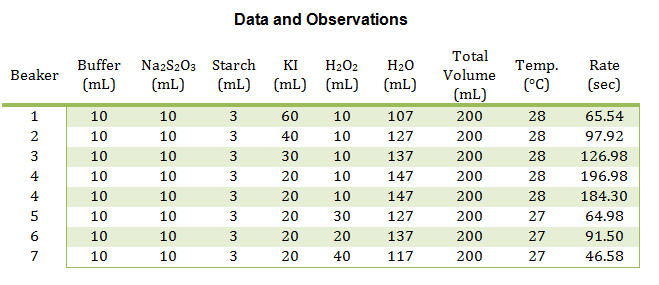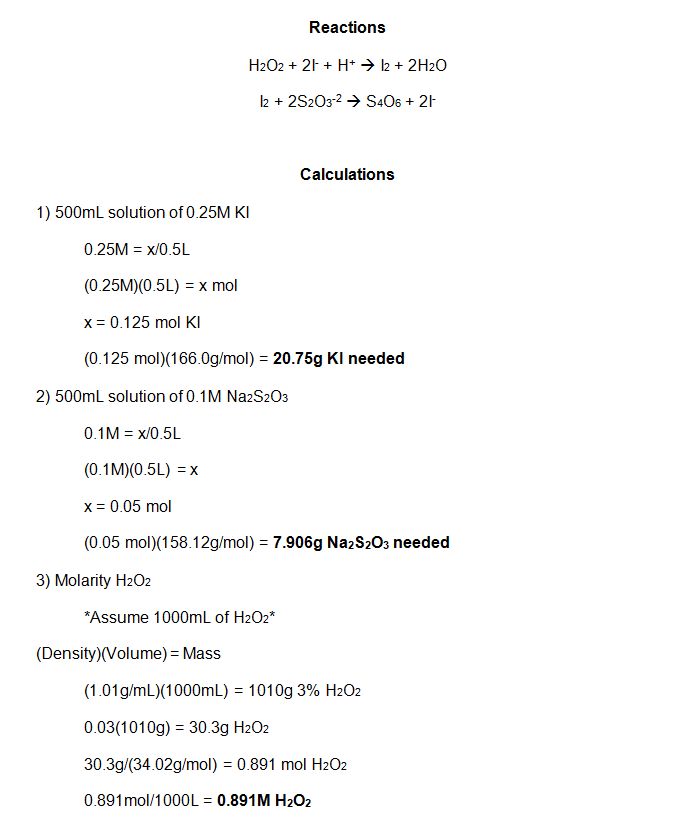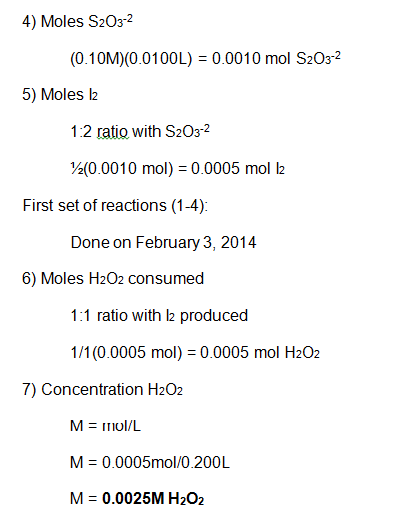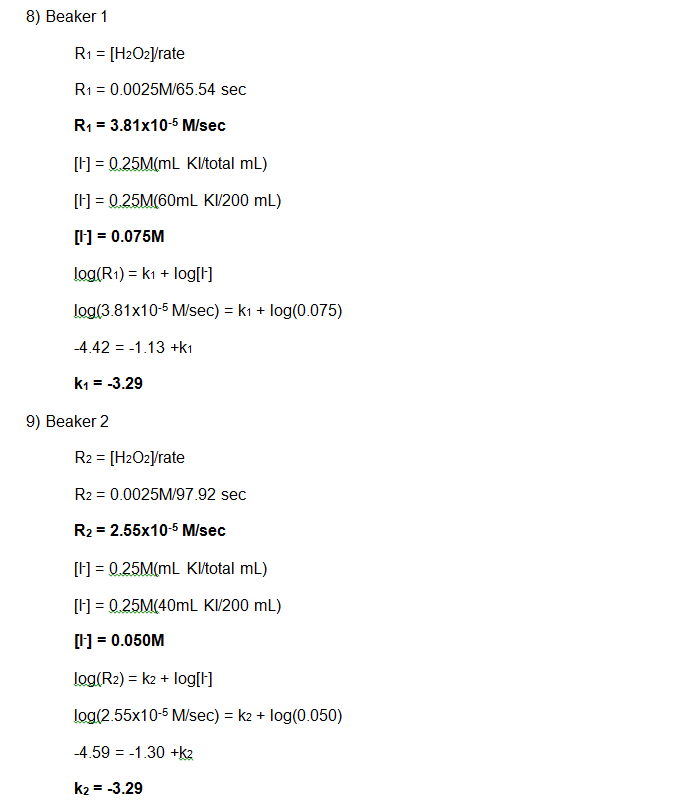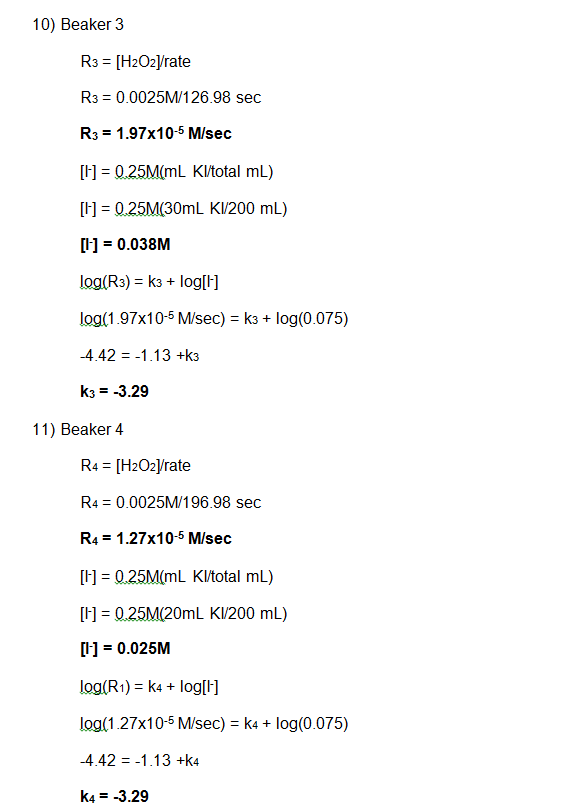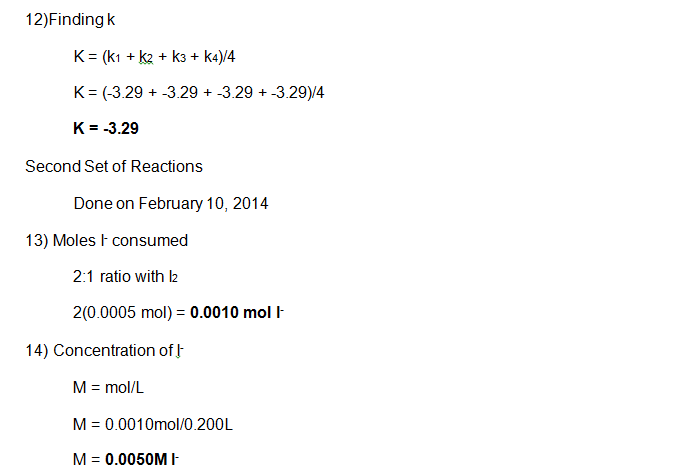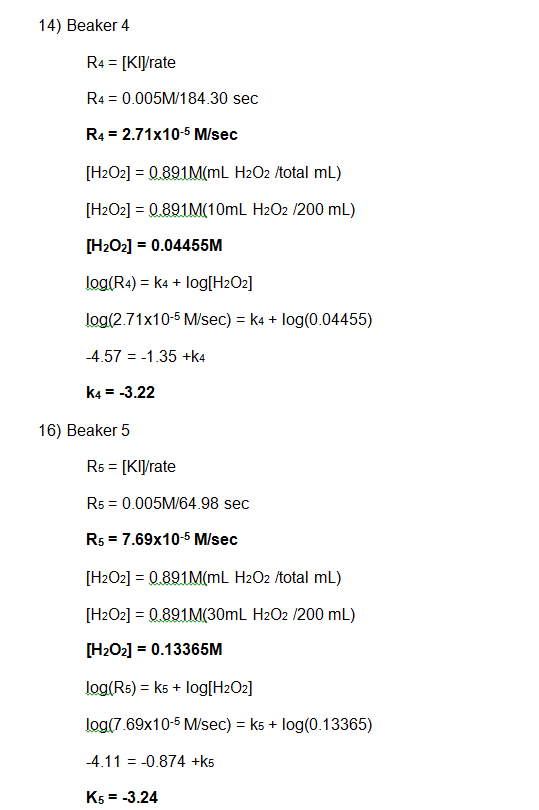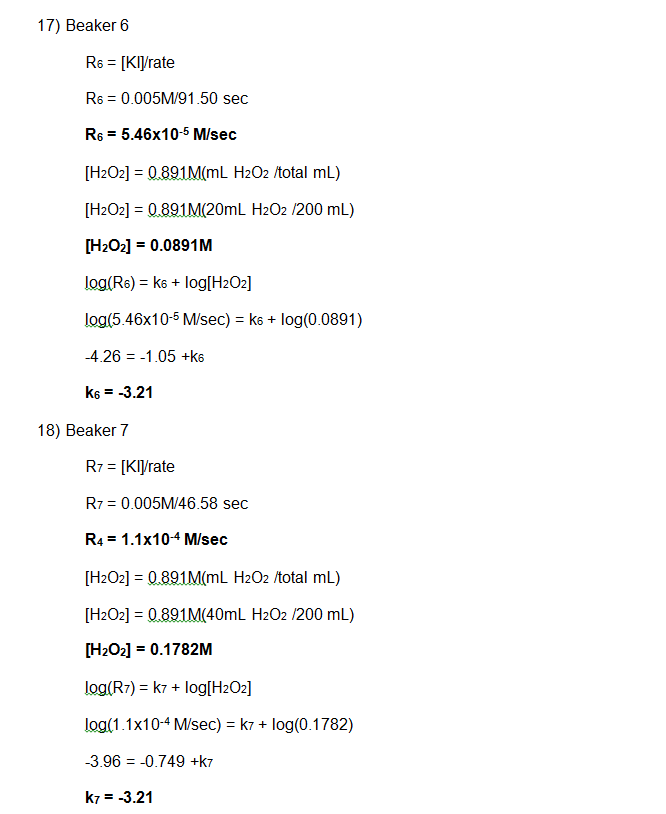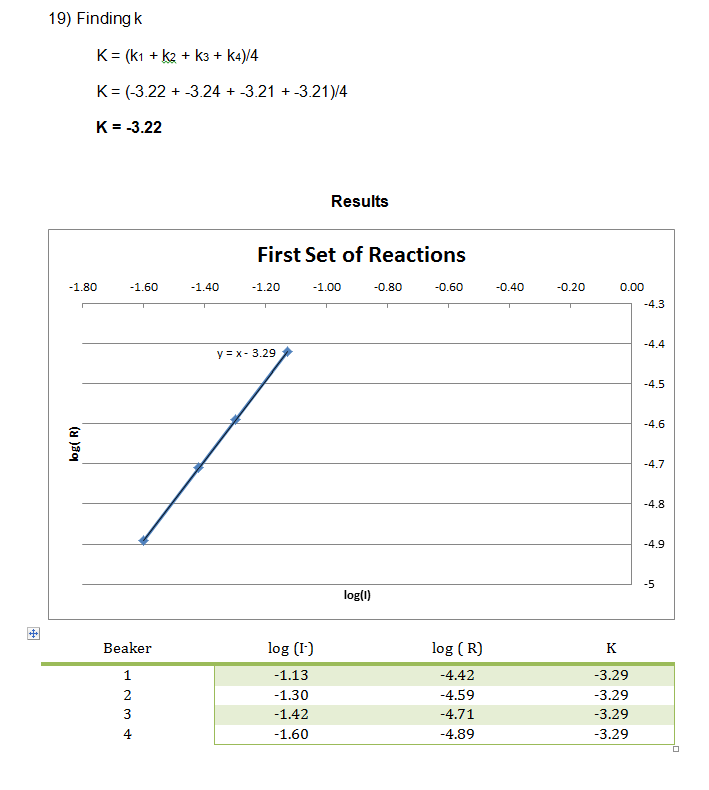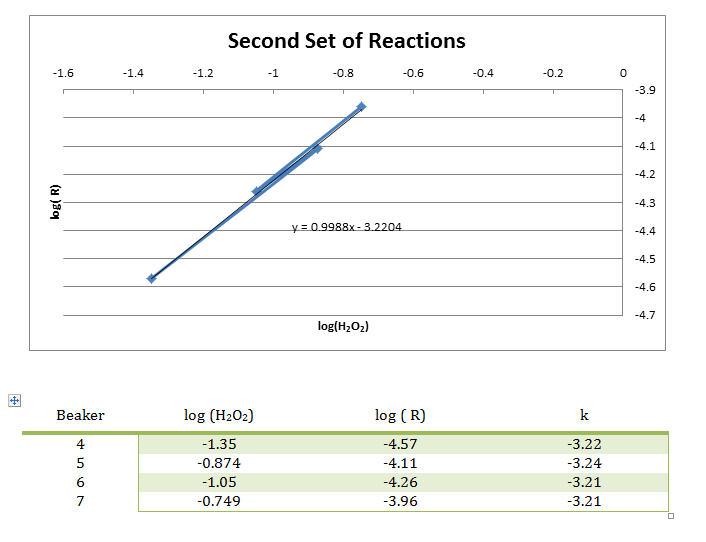Conclusion

In this experiment, numerous smaller reactions were carried out to demonstrate the chemical kinetics properties of rate laws.  During this experiment, the rate was determined by how long it took to create the color change.  This color change was from colorless to blue, then yellow/brown, which is from the reaction between the iodine and the starch, very similar to the color seen in iodine antiseptic wipes.  These reactions allowed the rate to be determined, and the rate constant to be evaluated from the graph of the log( R) v. log(I) and log( R) v. log(H2O2) respectively.  From this data, it can be concluded that the reaction has an overall order of 2 and that the I and H2O2 both have individual orders of 1.

References

1. Lecine, Ira N. 2002. Physical Chemistry. Fifth Edition.

2. Ngeyi, Stanley-Pierre, Ph.D. (2014) Physical Chemistry Notes. Madonna University. Livonia, MI

• Share